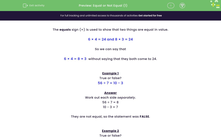# Equal or Not Equal (1)

In this worksheet, students must solve problems involving addition, subtraction, multiplication and division and a combination of these, including understanding the meaning of the equals sign.Key stage:  KS 2

Curriculum topic:   Number: Multiplication and Division

Popular topics:   Ratio worksheets

Difficulty level:#### Worksheet Overview

The equals sign (=) is used to show that two things are equal in value.

6 × 4 = 24 and 8 × 3 = 24

So we can say that

6 × 4 = 8 × 3  without saying that they both come to 24.

Example 1

True or false?

56 ÷ 7 = 10 - 3

Work out each side separately.

56 ÷ 7 = 8

10 - 3 = 7

They are not equal, so the statement was FALSE.

Example 2

True or false?

48 ÷ 4 = 4 × 3

Work out each side separately.

48 ÷ 4 = 12

4 × 3 = 12

They are equal, so the statement was TRUE.

### What is EdPlace?

We're your National Curriculum aligned online education content provider helping each child succeed in English, maths and science from year 1 to GCSE. With an EdPlace account you’ll be able to track and measure progress, helping each child achieve their best. We build confidence and attainment by personalising each child’s learning at a level that suits them.

Get started